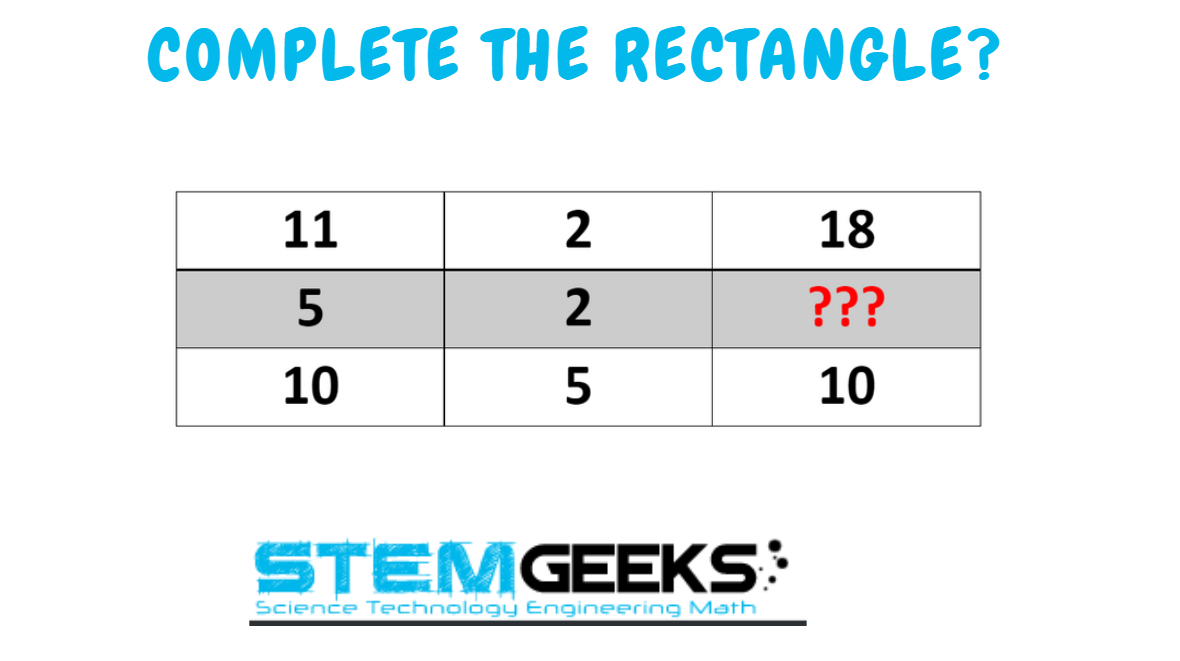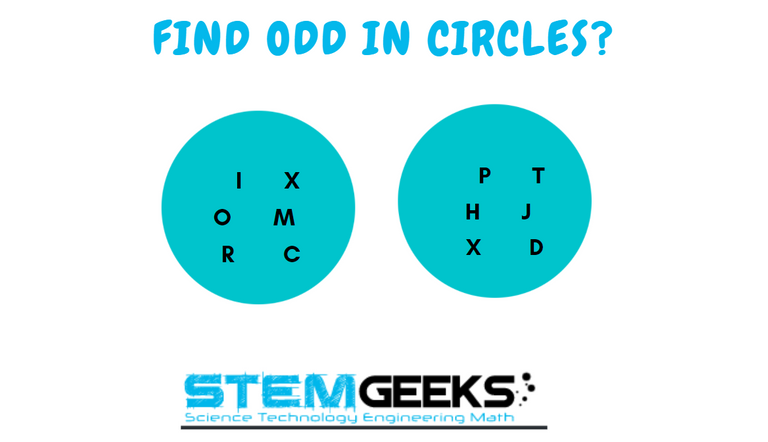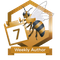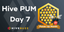# Maths Brain Teasers 117 :: Can You - Complete the Rectangle?

in STEMGeekslast year

Hey All; @StemGeeks Mathematician;

Without further, ado, let's get to our puzzle for today:: Maths Brain Teasers 117 :: Can You - Complete the Rectangle?Have a close look at the image given and see if you can find the correct number that replaces the question mark.

There is logic to resolve the puzzle; try finding out the logic and it should be resolved in seconds.. Guesswork isn't going to help...

With that, I'll leave you all with the Maths Brain Teasers 117 :: Can You - Complete the Rectangle? Good luck solving the puzzle...

##### Maths Brain Teasers 116 :: Can You - ODD Letter in the CIRCLES?- Solved with ExplanationLet's have a look at the Step by Step instructions to Solve the Maths Brain Teasers 116 :: Can You - ODD Letter in the CIRCLES?

If you carefully look at the Image in question; You would have noticed the following pattern is being followed::

The numerical values of each alphabet in circle no.1 are divisible by 3 and in circle no.2, the numbers are divisible by 4.

Now lets take some examples::

### M - numerical value is = 13 & 13 is not divisible by 3...

Therefore M is the odd one out in Circle No.1

In a similar fashion, let's see some examples from Circle No. 2

### J - numerical value is = 10 & 10 is not divisible by 4...

Therefore J is the odd one out in Circle No.2

Finally, the answer to the puzzle is Alphabets:: M && J

### STEM token GiveAway

I'll be again doing a giveaway of STEM tokens to the lucky random winner with the correct answer. For the last contest, which was Maths Brain Teasers 116 :: Can You - ODD Letter in the CIRCLES?.

We had 4 entries and I am happy to see the engagement. At the same time, it's great to see the detailed answers provided by everyone as to how they arrived at the solution of the puzzle.. Well done guys.. Way to GO... Now the results part; sorry to say that this time we don't have a winner. As none of the entries provided the right answer. The reward for this puzzle will be carried forwarded.

### Math Quote for the Day::

Here is the motivation to solve the Maths Equation Puzzle?If you like my work, then please spread the Word.. that we do have the Math Brain Teasers competition here @StemGeeks platform. Reblog is much appreciated.

Best RegardsFind Me on the Other Social Media Platforms::PS:: All the Maths Brain Teasers; are made by me using the Pro Canva License Version

Sort:

6?

5-2 x 2

(First Column - Second Column) × 2 = Third Column

1. 11-2 = 9×2 =18
2. 5-2 = 3×2 = 6
3. 10-5 = 5×2 = 10

Guess the right answer is 6.

11-2=9×2=18
5-2=3×2=6
10-5=5×2=10

The answer to the question is 6 which deals with subtracting 2 from five the multiply your answer by 2 which is 6
Ans=6

The last teaser was freaking tough. Whose mind would go there.. I would look at this week teaser and see if I can find the solution.

@tipu curate

last year Reveal Comment

Congratulations @gungunkrishu! You have completed the following achievement on the Hive blockchain and have been rewarded with new badge(s):You have been a buzzy bee and published a post every day of the week.

If you no longer want to receive notifications, reply to this comment with the word `STOP`Hive Power Up Month - Feedback from Day 7